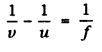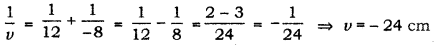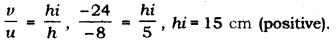# A 5 cm tall object is placed perpendicular to the principal axis of a convex lens of focal length 12 cm

A 5 cm tall object is placed perpendicular to the principal axis of a convex lens of focal length 12 cm. The distance of the object from the lens is 8 cm. Using the lens formula, find the position, size and nature of the image formed.

u = -8 cm, f = 12 cm, h = 5 cm
now lens formulaputting valuesThus image formed 24 cm on the left of the convex lens i.,e on the side of the object
Now magnificaction is m =